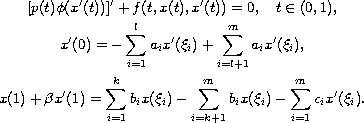Electron. J. Diff. Equ., Vol. 2010(2010), No. 22, pp. 1-20.

### Monotone positive solutions for p-Laplacian equations with sign changing coefficients and multi-point boundary conditions Jianye Xia, Yuji Liu

Abstract:
We prove the existence of three monotone positive solutions for the second-order multi-point boundary value problem, with sign changing coefficients,To obtain these results, we use a fixed point theorem for cones in Banach spaces. Also we present an example that illustrates the main results.

Submitted October 26, 2009. Published February 4, 2010.
Math Subject Classifications: 34B10, 34B15, 35B10.
Key Words: Second order differential equation; positive solution multi-point boundary value problem.

Show me the PDF file (281 KB), TEX file, and other files for this article.Jianye Xia Department of Mathematics Guangdong University of Finance Guangzhou 510320, China email: JianyeXia@sohu.com Yuji Liu Department of Mathematics Hunan Institute of Science and Technology Yueyang 414000, China email: liuyuji888@sohu.com